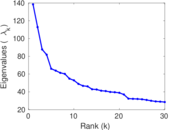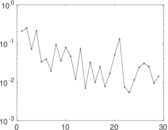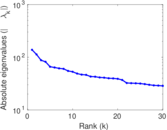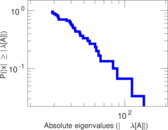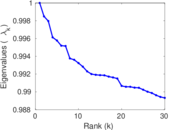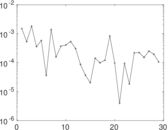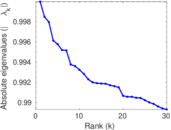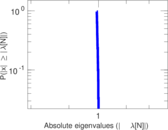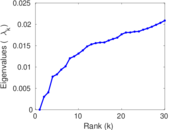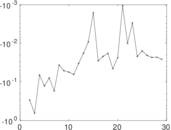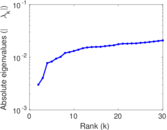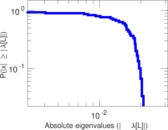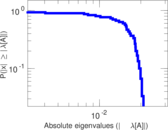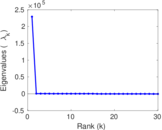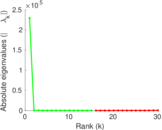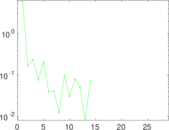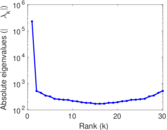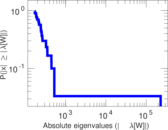# Occupations

This is the bipartite occupation network of DBpedia. It contains persons and occupations as nodes, and an edge denotes that a person has an occupation. The edges correspond to the <http://dbpedia.org/ontology/occupation> relationships in DBpedia.

 Code `OC` Internal name `dbpedia-occupation` Name Occupations Data source http://wiki.dbpedia.org/Downloads AvailabilityDataset is available for download Consistency checkDataset passed all tests Category Affiliation network Dataset timestamp 2001 ⋯ 2017 Node meaning Person, occupation Edge meaning Association Network formatBipartite, undirected Edge typeUnweighted, no multiple edges

## Statistics

 Size n = 229,307 Left size n1 = 127,577 Right size n2 = 101,730 Volume m = 250,945 Wedge count s = 335,154,441 Claw count z = 1,306,969,930,352 Cross count x = 4,967,920,242,807,365 Square count q = 24,509,245 4-Tour count T4 = 1,537,212,834 Maximum degree dmax = 17,997 Maximum left degree d1max = 24 Maximum right degree d2max = 17,997 Average degree d = 2.188 73 Average left degree d1 = 1.967 01 Average right degree d2 = 2.466 77 Fill p = 1.933 56 × 10−5 Size of LCC N = 143,220 Diameter δ = 24 50-Percentile effective diameter δ0.5 = 4.383 41 90-Percentile effective diameter δ0.9 = 6.770 30 Median distance δM = 5 Mean distance δm = 5.198 51 Gini coefficient G = 0.454 552 Balanced inequality ratio P = 0.347 200 Left balanced inequality ratio P1 = 0.392 126 Right balanced inequality ratio P2 = 0.287 824 Relative edge distribution entropy Her = 0.859 208 Power law exponent γ = 4.145 40 Tail power law exponent γt = 1.761 00 Tail power law exponent with p γ3 = 1.761 00 p-value p = 0.638 000 Left tail power law exponent with p γ3,1 = 5.161 00 Left p-value p1 = 0.000 00 Right tail power law exponent with p γ3,2 = 1.731 00 Right p-value p2 = 0.825 000 Degree assortativity ρ = +0.028 052 2 Degree assortativity p-value pρ = 7.149 25 × 10−45 Spectral norm α = 138.395 Algebraic connectivity a = 0.003 010 06 Spectral separation |λ1[A] / λ2[A]| = 1.226 83 Controllability C = 52,600 Relative controllability Cr = 0.229 393

## Plots

### Fruchterman–Reingold graph drawing### Degree distribution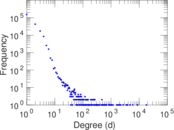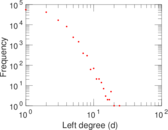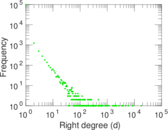### Cumulative degree distribution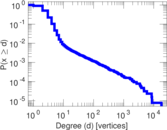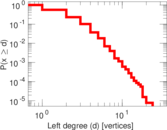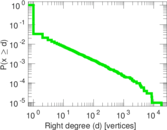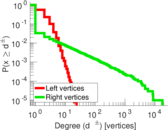### Lorenz curve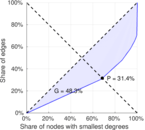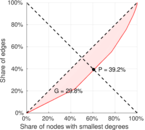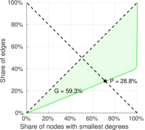### Spectral distribution of the adjacency matrix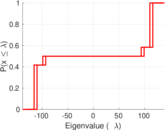### Spectral distribution of the normalized adjacency matrix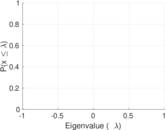### Spectral distribution of the Laplacian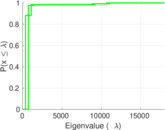### Spectral graph drawing based on the adjacency matrix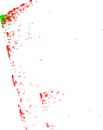### Spectral graph drawing based on the Laplacian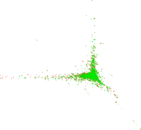### Spectral graph drawing based on the normalized adjacency matrix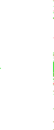### Degree assortativity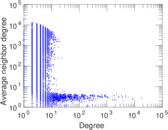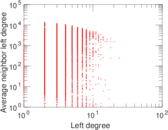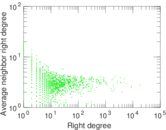### Zipf plot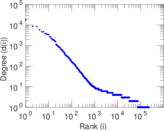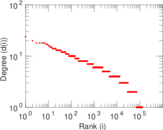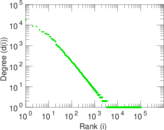### Hop distribution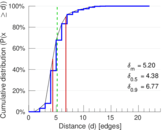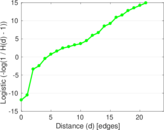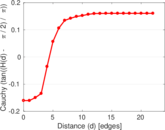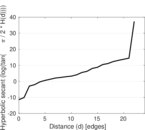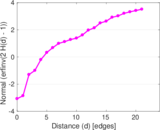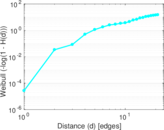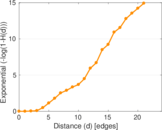### Delaunay graph drawing### Matrix decompositions plots